# Data Visualisation — How to Plot a Scatter Bubble Chart by Plotly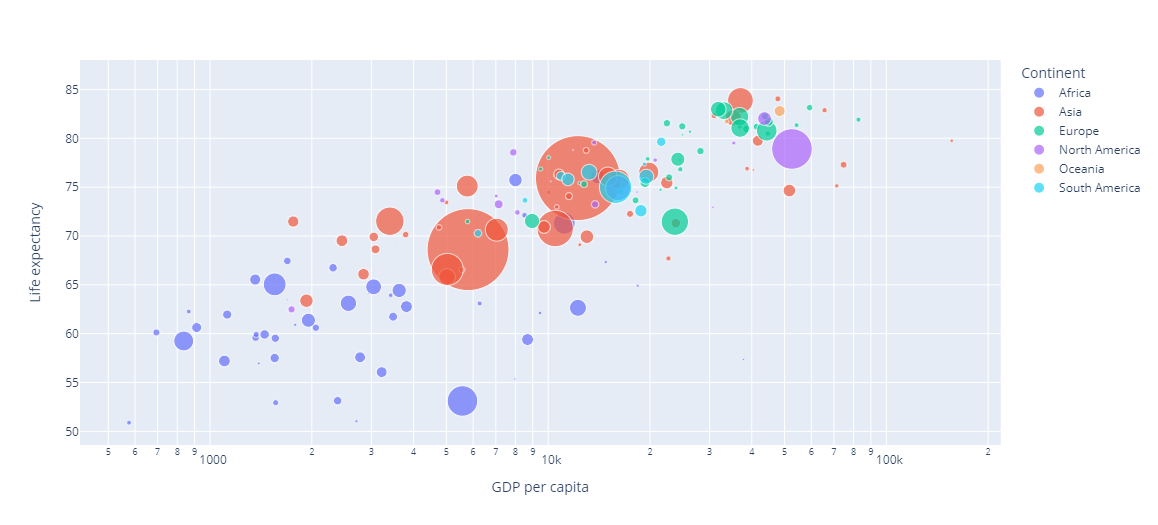Figure 1 A Scatter Bubble Chart of Life Expectancy versus GDP per capita (in log scale). Source of Data: Our World in Data
1. Install plotly and import plotly.express in Google Colab
`! pip install plotly==5.0.0import plotly.express as px`
`import pandas as pdfrom google.colab import drivedrive.mount('/content/drive/')data = pd.read_csv("drive/MyDrive/Colab Notebooks/life-expectancy-vs-gdp-per-capita.csv")data.head(5)`
`#define df2015 as the data of Year=2015df2015 = data.query("Year==2015")#plot the scatter plot by px of df2015 with x, y axis definedfig2015 = px.scatter(df2015, x="GDP per capita", y="Life expectancy")fig2015.show()`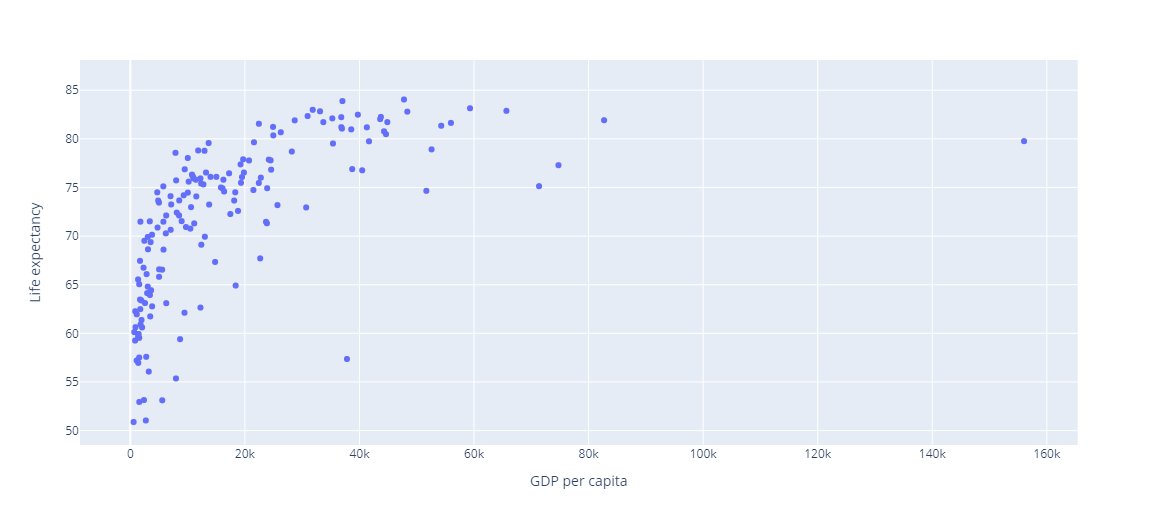Figure 2 A scatterplot of life expectancy versus GDP per capita.
`df2015 = data.query("Year==2015")#just add log_x=True to convert x-axis to log scalefig2015 = px.scatter(df2015, x="GDP per capita", y="Life expectancy", log_x=True)fig2015.show()`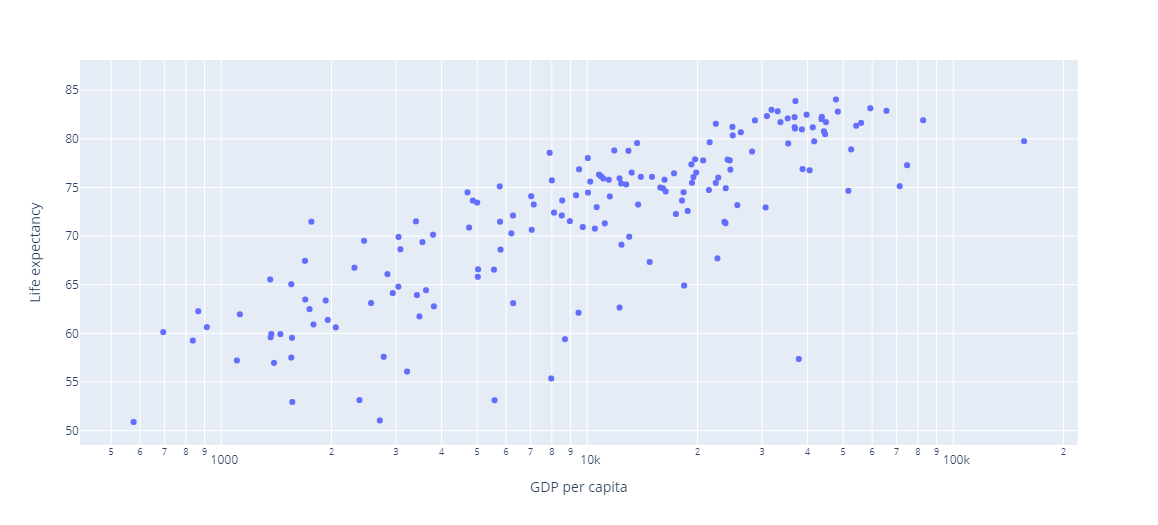Figure 3 A scatterplot of life expectancy versus GDP per capita in log scale — a stronger linear relationship is shown.
`df2015 = data.query("Year==2015")fig2015 = px.scatter(df2015, x="GDP per capita", y="Life expectancy", log_x=True, size="Total population", size_max=60, color="Continent", hover_name="Country")fig2015.show()`Figure 4 A scatter bubble chart of life expectancy versus GDP per capita in log scale — a stronger linear relationship is shown and the clusters of countries in different continents and their population sizes can easily be compared.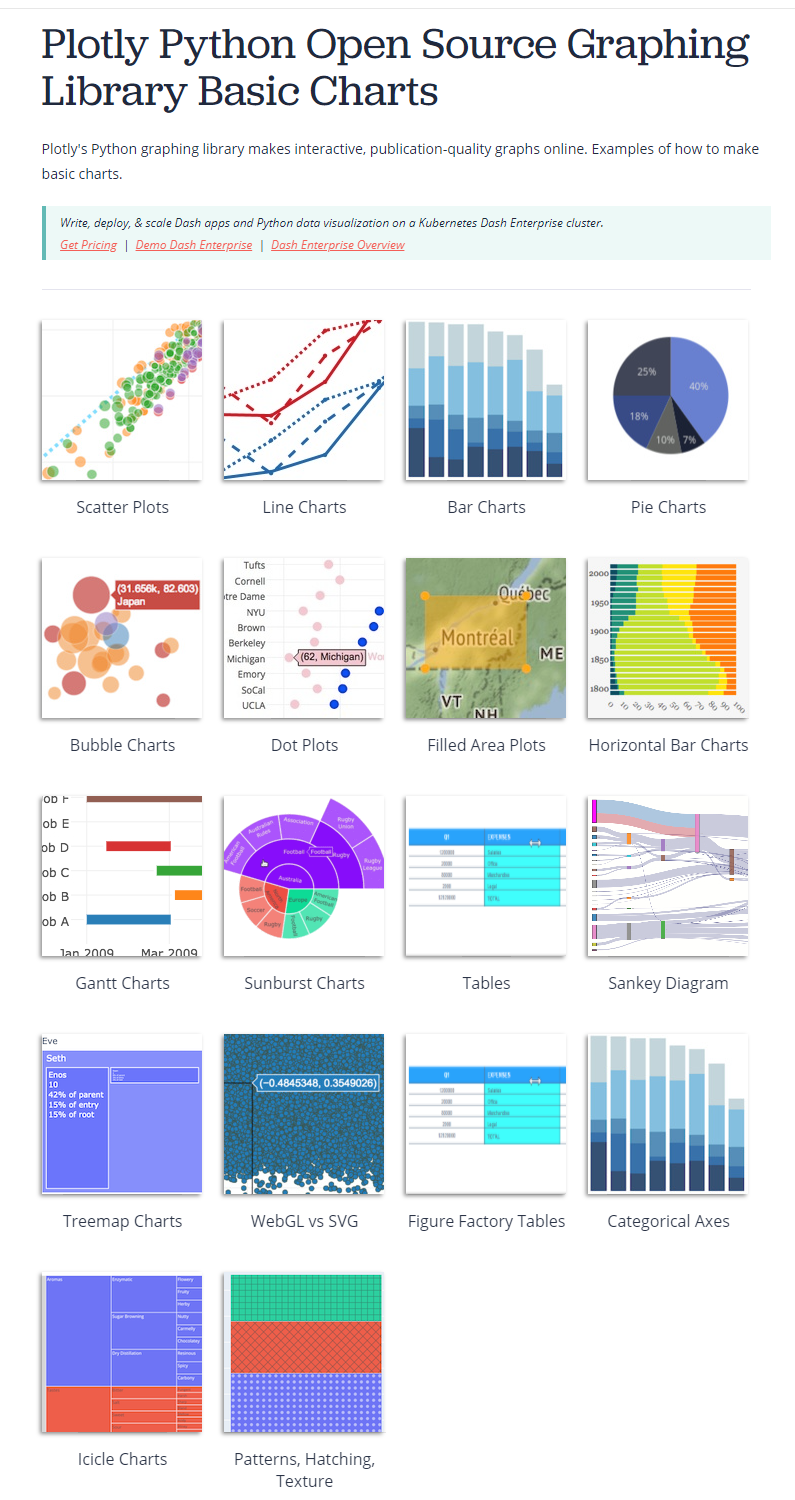Figure 5 Plotly Python Open Source graphing library basic charts. source https://plotly.com/python/basic-charts/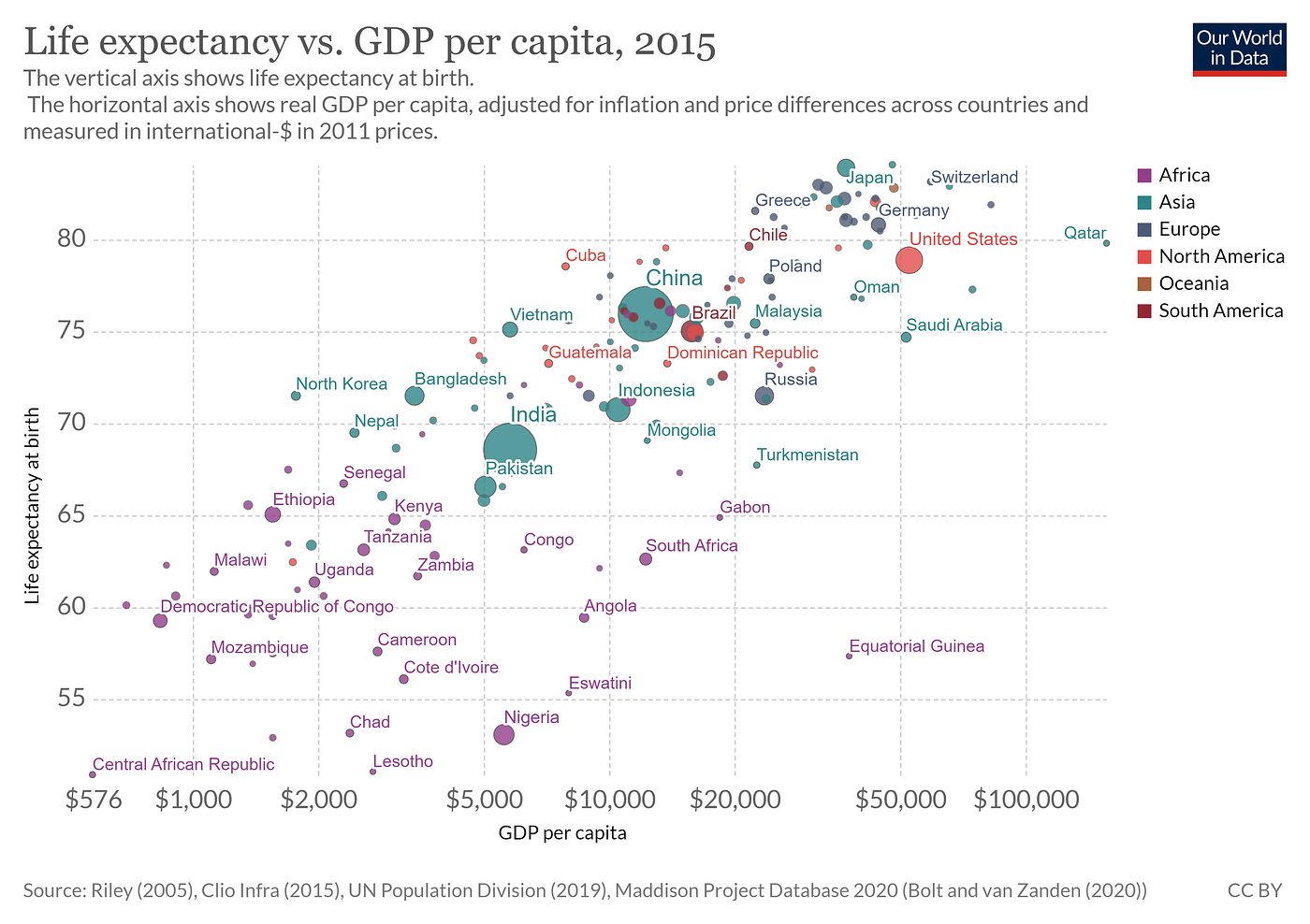Figure 6 The Scatter Bubble Chart provided by Our World in Data webpage. https://ourworldindata.org/grapher/life-expectancy-vs-gdp-per-capita

--

--

--

## More from ecyY

ecyY — easy to understand why, easy to study why. Finding the truths scientifically is the theme.

Love podcasts or audiobooks? Learn on the go with our new app.

## Visualizing and Representation of Stocks## Comparing Graph Databases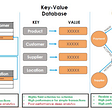## Weekly picks by Ali Alohali — مختارات اسبوعيه بواسطة علي العوهلي — Issue #28## Build Quick Models with Dabl## Bayesian Inference: Beyond Estimating Statistical Models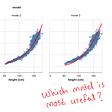## How to Create Interactive Plots with Altair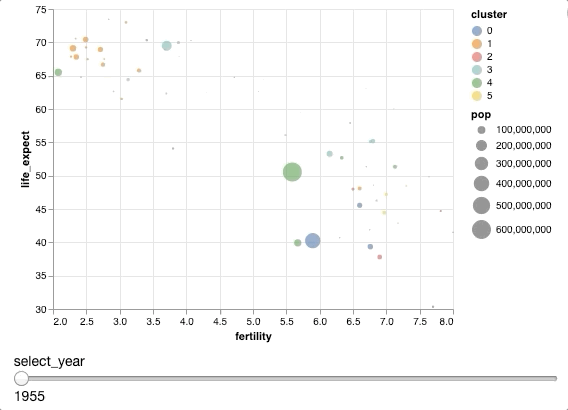## Data Analytics — Coding in Colab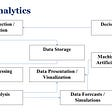## ecyY

ecyY — easy to understand why, easy to study why. Finding the truths scientifically is the theme.

## Collecting Historical Weather Data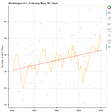## Beautiful plots with Seaborn## Plotting time series plot with matplotlib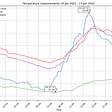## Building Pie Chart, Stacked Bar Chart & Column Bar Chart (With Data Labels) Using Matplotlib &…